# Python | Pandas DataFrame.abs()

Python is a great language for doing data analysis, primarily because of the fantastic ecosystem of data-centric python packages. Pandas is one of those packages and makes importing and analyzing data much easier.

`dataframe.abs()` is one of the simplest pandas dataframe function. It returns an object with absolute value taken and it is only applicable to objects that are all numeric. It does not work with any Nan value either. `abs()` function can also be used with complex numbers to find their absolute value.

For complex numbers, the absolute value is defined as: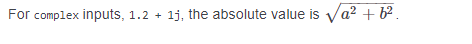```Syntax:  DataFrame.abs()
Returns: type of caller
```

Example #1: Replace team “Boston Celtics” with “Omega Warrior” in the nba.csv file

 `# importing pandas as pd ` `import` `pandas as pd ` ` `  `# Making data frame from the csv file ` `df ``=` `pd.read_csv(``"nba.csv"``) ` ` `  `# Printing the first 10 rows of the ` `# data frame for visualization ` `df[:``10``] `In order to find the absolute value, we also need to have negative values in the dataframe. So, let’s change some values to be negative for the demonstration purpose.

 `# This will set the Number column ` `# to be all negative. ` `df.Number ``=` `df.Number``*``(``-``1``) `

Output: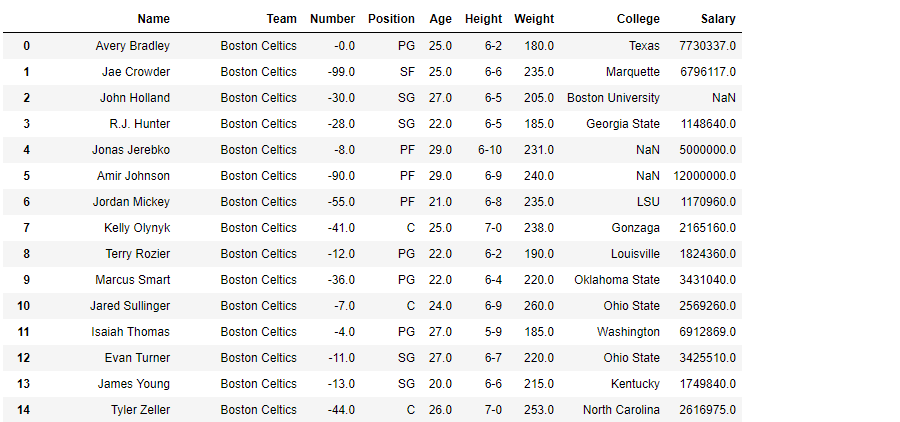Now let’s use` abs() `function to find only the absolute value of the Number column.

 `# Applying abs() value on one column only ` `df.Number.``abs``() `

Output: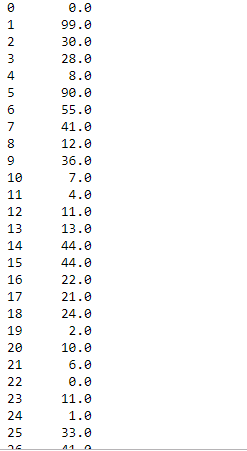Example #2: Applying `abs()` on a series with complex numbers.

 `# Importing pandas as pd ` `import` `pandas as pd ` ` `  `# Creating a series ` `ser ``=` `pd.Series([``1.2` `+` `1j``, ``2` `+` `5j``, ``1` `+` `8j``, ``3.2` `+` `2j``]) ` ` `  `# let's print the values in ser ` `ser `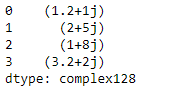`# Using abs() function to find the ` `# absolute value of the complex numbers ` `absolute_values ``=` `s.``abs``() ` ` `  `# Print the absolute values of all complex numbers ` `absolute_values `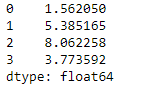My Personal Notes arrow_drop_upCheck out this Author's contributed articles.

If you like GeeksforGeeks and would like to contribute, you can also write an article using contribute.geeksforgeeks.org or mail your article to contribute@geeksforgeeks.org. See your article appearing on the GeeksforGeeks main page and help other Geeks.

Please Improve this article if you find anything incorrect by clicking on the "Improve Article" button below.

Article Tags :

Be the First to upvote.

Please write to us at contribute@geeksforgeeks.org to report any issue with the above content.